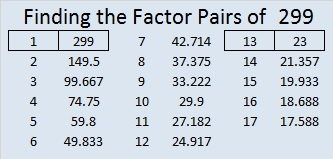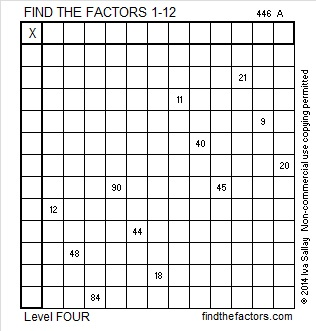# 299 and Level 4

• 299 is a composite number.
• Prime factorization: 299 = 13 x 23
• The exponents in the prime factorization are 1 and 1. Adding one to each and multiplying we get (1 + 1)(1 + 1) = 2 x 2 = 4. Therefore 299 has exactly 4 factors.
• Factors of 299: 1, 13, 23, 299
• Factor pairs: 299 = 1 x 299 or 13 x 23
• 299 has no square factors that allow its square root to be simplified. √299 ≈ 17.292Warning: The logic needed to solve this Level 4 puzzle is complicated.Print the puzzles or type the factors on this excel file: 12 Factors 2014-11-17This site uses Akismet to reduce spam. Learn how your comment data is processed.